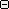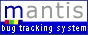Anonymous | Login | Signup for a new account 03-25-2019 03:16 PDT Project: All Projects Baratine Documentation Hessian Quercus Resin
 Main | My View | View Issues | Change Log | Docs

Viewing Issue Advanced Details Print ]
ID Category Severity Reproducibility Date Submitted Last Update
0002324 [Resin] minor always 01-14-08 08:56 03-05-08 17:06
Reporter ferg View Status public
Assigned To ferg
Priority normal Resolution fixed Platform
Status closed   OS
Projection none   OS Version
ETA none Fixed in Version 3.1.5 Product Version 3.1.4
Product Build
Summary 0002324: Hessian Calendar issue
Description (rep by tbee)

Ok, I'm quite baffled about this one. And it's not complex, just very very very strange. Bear with me.

Let me first give a quick description of the setup: I'm using Hessian 3.0.13 to communicate between an applet and a class running on Tomcat 4.1.31. Both the appplet and Tomcat run on Java 1.6.0_03. The applet calls a method with a GregorianCalendar as a parameter. The parameter is then cloned twice and one is moved to the beginning of the week, and the other to the end of the week. Then some more processing is done, but the problem is in the "beginning of the week part".

For example, if the passed date is "2006-01-08" then the first day in the week will be "2006-01-02". This is done by some fairly simple math using the Calendar's getFirstDayOfWeek() and get(Calendar.DAY_OF_WEEK) contained in the static gotoFirstDateOfWeek(c) utility method .

The fun part comes now.

If the gotoFirstDateOfWeek() method is called in the applet BEFORE the Calendar is sent using Hessian, this is the debug output:

gotoFirstDateOfWeek in=2006-01-08 19:49:38
!!!java.util.GregorianCalendar[time=1136746178453,areFieldsSet=true,areAllFieldsSet=true,lenient=true,zone=sun.util.calendar.ZoneInfo[id="Europe/Berlin",offset=3600000,dstSavings=3600000,useDaylight=true,transitions=143,lastRule=java.util.SimpleTimeZone[id=Europe/Berlin,offset=3600000,dstSavings=3600000,useDaylight=true,startYear=0,startMode=2,startMonth=2,startDay=-1,startDayOfWeek=1,startTime=3600000,startTimeMode=2,endMode=2,endMonth=9,endDay=-1,endDayOfWeek=1,endTime=3600000,endTimeMode=2]],firstDayOfWeek=2,minimalDaysInFirstWeek=4,ERA=1,YEAR=2006,MONTH=0,WEEK_OF_YEAR=1,WEEK_OF_MONTH=1,DAY_OF_MONTH=8,DAY_OF_YEAR=8,DAY_OF_WEEK=1,DAY_OF_WEEK_IN_MONTH=2,AM_PM=1,HOUR=7,HOUR_OF_DAY=19,MINUTE=49,SECOND=38,MILLISECOND=453,ZONE_OFFSET=3600000,DST_OFFSET=0]
!!!1.6.0_03
lFirstDayOfWeek=2
lCurrentDayOfWeek=1
delta=-6
gotoFirstDateOfWeek out=2006-01-02 19:49:38
!!!java.util.GregorianCalendar[time=1136230848015,areFieldsSet=true,areAllFieldsSet=true,lenient=true,zone=sun.util.calendar.ZoneInfo[id="Europe/Berlin",offset=3600000,dstSavings=3600000,useDaylight=true,transitions=143,lastRule=java.util.SimpleTimeZone[id=Europe/Berlin,offset=3600000,dstSavings=3600000,useDaylight=true,startYear=0,startMode=2,startMonth=2,startDay=-1,startDayOfWeek=1,startTime=3600000,startTimeMode=2,endMode=2,endMonth=9,endDay=-1,endDayOfWeek=1,endTime=3600000,endTimeMode=2]],firstDayOfWeek=2,minimalDaysInFirstWeek=4,ERA=1,YEAR=2006,MONTH=0,WEEK_OF_YEAR=1,WEEK_OF_MONTH=1,DAY_OF_MONTH=2,DAY_OF_YEAR=2,DAY_OF_WEEK=2,DAY_OF_WEEK_IN_MONTH=1,AM_PM=1,HOUR=8,HOUR_OF_DAY=20,MINUTE=40,SECOND=48,MILLISECOND=15,ZONE_OFFSET=3600000,DST_OFFSET=0]

Perfect! You can see the incoming date, the fact that 6 days need to be substracted and the resulting date.

Now the calendar is sent over the line and the same method is called in the class:

gotoFirstDateOfWeek in=2006-01-08 19:49:38
!!!java.util.GregorianCalendar[time=1136746178453,areFieldsSet=true,areAllFieldsSet=true,lenient=true,zone=sun.util.calendar.ZoneInfo[id="Europe/Berlin",offset=3600000,dstSavings=3600000,useDaylight=true,transitions=143,lastRule=java.util.SimpleTimeZone[id=Europe/Berlin,offset=3600000,dstSavings=3600000,useDaylight=true,startYear=0,startMode=2,startMonth=2,startDay=-1,startDayOfWeek=1,startTime=3600000,startTimeMode=2,endMode=2,endMonth=9,endDay=-1,endDayOfWeek=1,endTime=3600000,endTimeMode=2]],firstDayOfWeek=2,minimalDaysInFirstWeek=4,ERA=1,YEAR=2006,MONTH=0,WEEK_OF_YEAR=1,WEEK_OF_MONTH=1,DAY_OF_MONTH=8,DAY_OF_YEAR=8,DAY_OF_WEEK=1,DAY_OF_WEEK_IN_MONTH=2,AM_PM=1,HOUR=7,HOUR_OF_DAY=19,MINUTE=49,SECOND=38,MILLISECOND=453,ZONE_OFFSET=3600000,DST_OFFSET=0]
lFirstDayOfWeek=2
lCurrentDayOfWeek=1
delta=-6
gotoFirstDateOfWeek out=2008-01-04 19:49:38
!!!java.util.GregorianCalendar[time=1199475648015,areFieldsSet=true,areAllFieldsSet=true,lenient=true,zone=sun.util.calendar.ZoneInfo[id="Europe/Berlin",offset=3600000,dstSavings=3600000,useDaylight=true,transitions=143,lastRule=java.util.SimpleTimeZone[id=Europe/Berlin,offset=3600000,dstSavings=3600000,useDaylight=true,startYear=0,startMode=2,startMonth=2,startDay=-1,startDayOfWeek=1,startTime=3600000,startTimeMode=2,endMode=2,endMonth=9,endDay=-1,endDayOfWeek=1,endTime=3600000,endTimeMode=2]],firstDayOfWeek=2,minimalDaysInFirstWeek=4,ERA=1,YEAR=2008,MONTH=0,WEEK_OF_YEAR=1,WEEK_OF_MONTH=1,DAY_OF_MONTH=4,DAY_OF_YEAR=4,DAY_OF_WEEK=6,DAY_OF_WEEK_IN_MONTH=1,AM_PM=1,HOUR=8,HOUR_OF_DAY=20,MINUTE=40,SECOND=48,MILLISECOND=15,ZONE_OFFSET=3600000,DST_OFFSET=0]

A totally different outcome! Look at the year! And it still only substracted 6 days. To answer the cry for sourcecode:

static public Calendar gotoFirstDateOfWeek(Calendar c)
{
System.out.println( "gotoFirstDateOfWeek in=" + quickFormatCalendar(c));
System.out.println( "!!!" + c);
System.out.println( "!!!" + System.getProperty("java.version"));

int lFirstDayOfWeek = c.getFirstDayOfWeek();
System.out.println( "lFirstDayOfWeek=" + lFirstDayOfWeek);
int lCurrentDayOfWeek = c.get(Calendar.DAY_OF_WEEK);
System.out.println( "lCurrentDayOfWeek=" + lCurrentDayOfWeek);
int lDelta = 0;
if (lFirstDayOfWeek <= lCurrentDayOfWeek)
{
lDelta = -lCurrentDayOfWeek + lFirstDayOfWeek;
}
else
{
lDelta = -lCurrentDayOfWeek - (7-lFirstDayOfWeek);
}
System.out.println( "delta=" + lDelta);
System.out.println( "gotoFirstDateOfWeek out=" + quickFormatCalendar(c));
System.out.println( "!!!" + c);
return c;
}

Now, I can actually work around this, by inserting the following code at the beginning of the method:

static public Calendar gotoFirstDateOfWeek(Calendar c)
{
Calendar o = c;
c = new GregorianCalendar();
c.setFirstDayOfWeek( o.getFirstDayOfWeek() );
c.setMinimalDaysInFirstWeek( o.getMinimalDaysInFirstWeek() );
c.setTimeInMillis( o.getTimeInMillis() );

System.out.println( "gotoFirstDateOfWeek in=" + quickFormatCalendar(c));
...
}

This basically creates a new Calendar and sets all relevant properties so the behavior is correct again. Cloning does not help, so something must get screwed when sending a Calendar via Hessian.

I tried the 3.1.3 release, but I get a bunch of HTTP 500 errors, so no luck there so far.

Any suggestions?

Steps To ReproduceRelationshipsIssue History Date Modified Username Field Change 01-14-08 08:56 ferg New Issue 01-14-08 09:13 ferg Project Quercus => Resin 03-05-08 17:06 ferg Assigned To => ferg 03-05-08 17:06 ferg Status new => closed 03-05-08 17:06 ferg Resolution open => fixed 03-05-08 17:06 ferg Fixed in Version => 3.1.5
 Copyright © 2000 - 2005 Mantis Group 26 total queries executed. 24 unique queries executed.